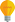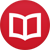# Calculus of finite differences without variables

by Jakub MarianTip: See my list of theMost Common Mistakes in English. It will teach you how to avoid mis­takes with com­mas, pre­pos­i­tions, ir­reg­u­lar verbs, and much more.

This article contains an elementary introduction to calculus of finite differences. Instead of working with a variable, say $n$, it uses a different approach based on my article about definition of functions without using variables. Just to remind you: $\id$ denotes the identity function $\id(x) = x$. Let’s start with an elementary definition:

The falling factorial $x^{\underline n,h}$ is defined as the following product of $n$ terms:
$$x^{{\underline n},h} = x(x-h)\cdots(x-(n-1)h)\,,$$
pronounced “$x$ to the $n$ falling by $h$”. In particular, we define $x^{\underline n} = x^{\underline{n},1}$, which is pronounced “$x$ to the $n$ falling”.

The notation defined above gives rise to the $n^\mathrm{th}$ falling factorial function $\id^{\underline n}$, meaning $\id^{\underline n}(x) = x^{\underline n}$. This function plays the same role in the discrete theory as $\id^n$ does in the smooth theory, which will become apparent after the next definition.

Let $f$ be a function. The difference operators $Δ_h$ are defined for $h ≠ 0$ by $$(Δ_hf)(x) = ÷{1}{h}\big(f(x+h)-f(x)\big)\,,$$ or, without the variable $$Δ_hf = ÷{1}{h}\big(f(\id+h)-f\big)\,.$$ In particular, we define $Δ = Δ_1$. Note: The symbol $Δ_h$ is commonly used for another operator which would be written as $hΔ_h$ in our notation.

Notice how $Δ_hf$ approximates the derivative of $f$ as $h → 0$. As we will see, $Δ_h$ has some nice algebraic properties which translate trivially into properties of derivatives after taking the limit for $h → 0$.

It is a simple exercise to check that

$$Δ_h(\id^{\underline n,h}) = n\id^{\underline {n-1},h}\,.$$

In particular,

$$Δ(\id^{\underline n}) = n\id^{\underline{n-1}}\,.$$

Just to demonstrate how to work without variables, we’ll prove the latter formula:

\begin{align*} Δ\id^{\underline n} &= \id^{\underline n}∘(\id+1)-\id^{\underline n} = \big(\id(\id-1)\cdots(\id-n+1)\big) ∘ (\id+1)-\id^{\underline n}\\ &= (\id+1)\id(\id-1)\cdots(\id-n+2)-\id(\id-1)\cdots(\id-n+1) \\ &= (\id+1-\id+n-1)\id(\id-1)\cdots(\id-n+2) = n\id^{\underline{n-1}}\,. \end{align*}

The difference operator is obviously linear, i.e. for $f,g$ functions and $a,b ∈ℝ$, $Δ(af+bg) = aΔf + bΔg$. Notice that the binomial coefficient can be expressed as

$$\binom nk = ÷{n^{\underline k}}{k!}$$

Using linearity and the formula above, we can derive a nice formula for $Δ\binom \id k$:

$$Δ\binom \id k = Δ\l(÷{\id^{\underline k}}{k!}\r) = ÷{1}{k!}k\id^{\underline{k-1}} = ÷{\id^{\underline{k-1}}}{(k-1)!} = \binom \id{k-1}\,.$$

The role of the exponential in the discrete theory is played by the function $2^\id$, for which we have:

$$Δ2^\id = 2^{\id}∘(\id+1)-2^\id = 2^{\id+1}-2^\id = 2⋅2^\id-2^\id = 2^\id$$

Of course, everything we have done so far can be rewritten with variables using the notion of application of an operator with respect to a variable defined in a previous article. We could have written, for example

$$\underset {n\vphantom{h}}Δ\binom nk = \underset nΔ\l(÷{n^{\underline k}}{k!}\r) = ÷{1}{k!}kn^{\underline{k-1}} = ÷{n^{\underline{k-1}}}{(k-1)!} = \binom n{k-1}\,.$$

The following theorem shows that finite differences have a similar algebraic structure as derivatives:

Let $f$ and $g$ be functions. The difference operator satisfies the following product rule: $$Δ_h(fg) = fΔ_hg+gΔ_hf+h(Δ_hf)(Δ_hg)\,,$$ and the quotient rule: $$Δ_h\l(÷{f}{g}\r) = ÷{gΔ_hf-fΔ_hg}{g^2+hgΔ_hg}\,.$$

Notice how these become exactly the well known rules for derivatives after applying $\lim_{h→0}$. What is lacking in the discrete theory and what makes the smooth theory so much more practical to work with is the chain rule; there is no nice general expression for $Δ_h(f(g))$.

By the way, I have written several educational ebooks. If you get a copy, you can learn new things and support this website at the same time—why don’t you check them out?